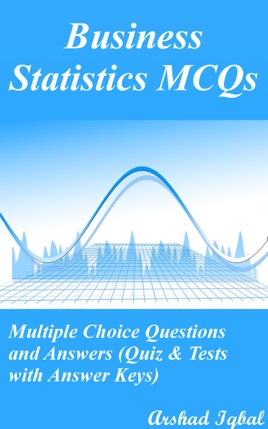• 3,99 €

## Descrizione dell’editore

Business Statistics Multiple Choice Questions and Answers pdf: MCQs, Quizzes & Practice Tests. Business statistics quiz questions and answers pdf with practice tests for online exam prep and job interview prep. Business statistics study guide with questions and answers about confidence intervals and estimation, data classification, tabulation and presentation, introduction to probability, introduction to statistics, measures of central tendency, measures of dispersion, probability distributions, sampling distributions, skewness, kurtosis and moments.
Business statistics MCQ questions and answers to get prepare for career placement tests and job interview prep with answers key. Practice exam questions and answers about statistics, composed from Business statistics textbooks on chapters:

Confidence Intervals and Estimation Practice Test: 21 MCQs
Data Classification, Tabulation and Presentation Practice Test: 65 MCQs
Introduction to Probability Practice Test: 64 MCQs
Introduction to Statistics Practice Test: 64 MCQs
Measures of Central Tendency Practice Test: 71 MCQs
Measures of Dispersion Practice Test: 97 MCQs
Probability Distributions Practice Test: 83 MCQs
Sampling Distributions Practice Test: 53 MCQs
Skewness, Kurtosis and Moments Practice Test: 58 MCQs

Business statistics interview questions and answers on arithmetic mean, average deviation measures, averages of position, bayes theorem, binomial distribution, binomial probability distribution, calculating moments, central tendency measures, chebyshev theorem, class width in statistics, classification: measures of dispersion, cluster sampling, coefficient of skewness, comparison: measures of central tendency, confidence interval estimation, continuous probability distribution.
Business statistics test questions and answers on data classification, data measurement in statistics, data tables and types, data tables in statistics, data types in stats, definition of probability, discrete probability distributions, distance measures, empirical values, expected value and variance, exponential distribution, frequency curve, frequency distribution types, harmonic mean, histograms in statistics, hyper geometric distribution, interquartile deviation, interquartile range of deviation, introduction of estimation.
Business statistics exam questions and answers on introduction to statistics, kurtosis in business statistics, learning business statistics, mean absolute deviation, measurements in statistics, measures of skewness, measuring dispersion, median, mean and mode, multiplication rules of probability, normal distribution, percentiles, Poisson distribution, population parameters and sample statistic.
Business statistics objective questions and answers on principles of measurement, principles of sampling, probability and counting rules, probability experiments, probability rules, random variable classes, rectangular distribution, relationship: measures of deviation, relationship: median, mode and mean, relative frequency, relative measure of skewness, rules of probability and algebra, sample space, sample statistics, sampling distribution in statistics, sampling distributions, sampling techniques.
Business statistics certification questions on skewness and skewed distribution, sources of data, squared deviation, standard deviation in stats, standard errors in statistics, standard normal probability distribution, statistical analysis methods, statistical data analysis, statistical measures, statistical techniques, statistics formulas, statistics questions answers, stratified sampling, structured data, symmetrical distribution, types of bias, types of events, types of statistical methods, uniform distribution, variance and standard deviation, variance in statistics for competitive exams preparation.